# SSC JE Electrical Important MCQ Part 26

SSC JE Electrical Important MCQ PDF Part 26

1.Find voltage vin the circuit shown in Fig.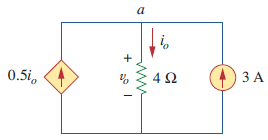A.4 V

B.24 V

C.28 V

D.6 V

2.The current through an element is shown in Fig. What is the total charge that passed through the element at t=3 s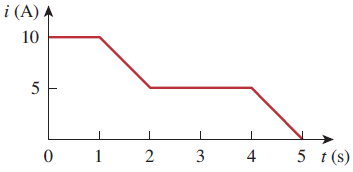A.10 C

B.22.5C

C.30 C

D.120 mC

3.The charge entering a certain element is shown in Fig.The value of current at t = 6 ms is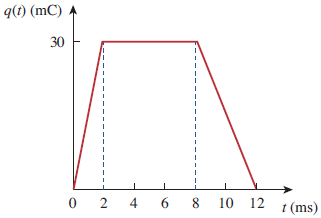A.30 A

B.0 A

C.-15 A

D.15 A

Details Solution Available In Electrical One Year Test Series Pass

4.A lightning bolt with 10 kA strikes an object for 15 s. How much charge is deposited on the object

A.120 mC

B.120 C

C.12 mC

D.12 C

5.Figure shows a circuit with five elements. If P1=-205 W, P2=60 W, P4=45 W, P5= 30 W,What is the power received or delivered by element 3A.70 W

B.-70 W

C.30 W

D.-30 W

6.A network with b branches, n nodes, and l independent loops will satisfy the which of following fundamental theorem of network topology

A.b = l + n – 1

B.b = l + n + 1

C.b = l – n – 1

D.b =- l + n + 1

7.Which of following equation will be satisfy KVL of circuit given in figure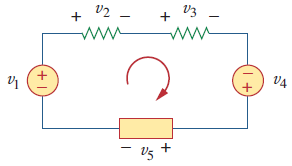A.v2 + v3 + v5 = v1 + v4

B.v2 + v3 + v5 = v1 – v4

C.v2 + v3 – v5 = v1 + v4

D.v2 – v3 – v5 = v1 + v4

8.Find Req for the circuit shown in Fig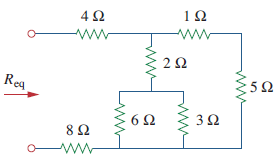A.14.4 Ω

B.2.4 Ω

C.10.4 Ω

D.12.4 Ω

Details Solution Available In Electrical One Year Test Series Pass

9.In the circuit of Fig. If R3 decrease then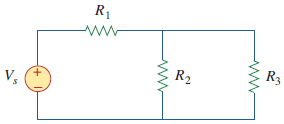(a) current through R3 decrease
(b) voltage across R3 decrease
(c) voltage across R1 decrease
(d) power dissipated in R2 decrease

Which is true

A.a,d

B.b,d

C.a,b,d

D.a,b,c,d

10.A network has 12 branches and 8 independent loops. How many nodes are there in the network

A.19

B.17

C.5

D.4

11.Value of current i in Fig. when the switch is in position 1.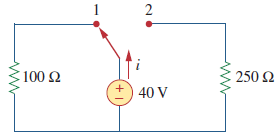A.25 mA

B.4 mA

C.40 mA

D.0 mA

12.For large values of ∣VDS∣, a FET behaves as a

A.voltage-controlled resistor

B.current-controlled current source

C.voltage-controlled current source

D.current-controlled resistor

13.In MOSFET devices, the N-channel type is better than the P-channel type in the following respect

A.It has better noise immunity

B.It is faster

C.It is TTL compatible

D.It has better drive capability

14.In a MOSFET, the polarity of the inversion layer is the same as that of the

A.majority carriers in the drain

B.minority carries in the drain

C.majority carries in the substrate

D.majority carries in the source

Details Solution Available In Electrical One Year Test Series Pass

15.A BJT is said to be operating in the saturation region if

A.both the junctions are reverse biased

B.base—emitter junction is reverse biased and base collector junction is forward biase

C.base—emitter junction is forward biased and base—collector junction reverse biased

D.both the junctions are forward biased

16.The early-effect in a BJT is caused by

A.fast turn-on

B.fast turn-off

C.large collector—base reverse bias

D.large emitter—base forward bias

17.According to Hall effect, the Hall voltage is proportional to—–(B is the magnetic field and I is the current.)

A.the product of B and I

B.inverse of the product of B and I

C.I only

D.B only

18.The unit of q/kT is

A.V

B.V-1

C.J

D.J/K

19.Drift current in semiconductors depends upon

A.only the electric field

C.both the electric field and the carrier concentration

D.both the electric field and the carrier concentration gradient

20.The diffusion potential across a P—N junction

A.decreases with increasing doping concentration

B.increases with decreasing band gap

C.does not depend on doping concentrations

D.increases with increase in doping concentration